Courses

# Integration by Reduction Formulae - Indefinite Integration, Class 12, Maths Class 12 Notes | EduRev

## Class 12 : Integration by Reduction Formulae - Indefinite Integration, Class 12, Maths Class 12 Notes | EduRev

The document Integration by Reduction Formulae - Indefinite Integration, Class 12, Maths Class 12 Notes | EduRev is a part of Class 12 category.
All you need of Class 12 at this link: Class 12

F. INTEGRATION BY REDUCTION FORMULAE

Ex.47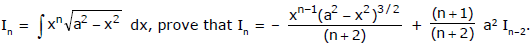Sol.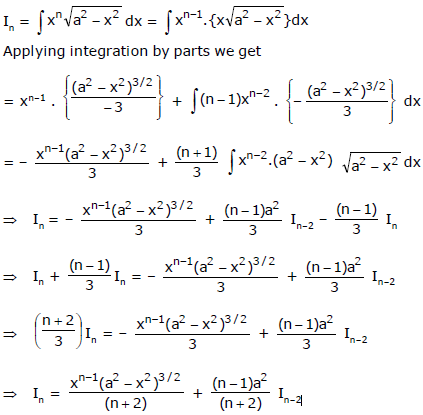Ex.48 Integration of 1/(x2 + k)n.

Sol.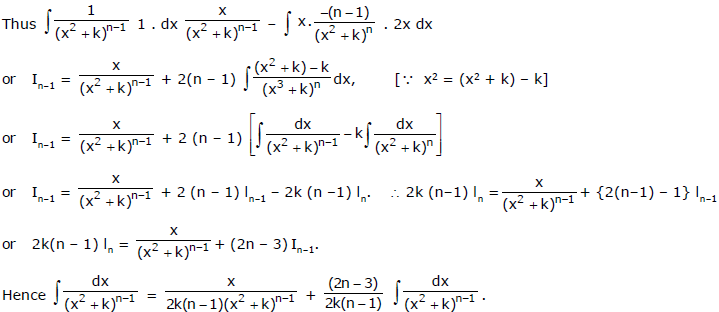Above is the reduction formula for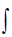[1/(x2 + k)n]dx . By repeated application of this formula the integral shall reduce to that of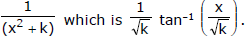Ex.49 Integrate (x + 2) / (2x2 + 4x + 3)2.

Sol. Here (d/dx) (2x2 + 4x + 3) = 4x + 4.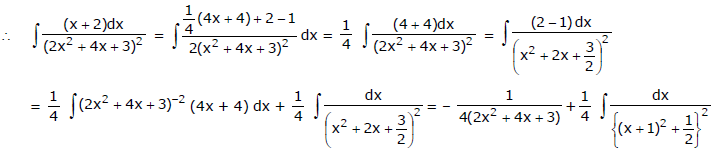Now put x + 1 = t and then applying the reduction formula, we get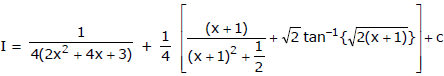Ex.50 Integrate (2x + 3)/(x2 + 2x + 3)2.

Sol. Here (d/dx) (x2 + 2x + 3) = 2x + 2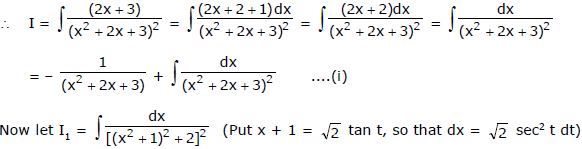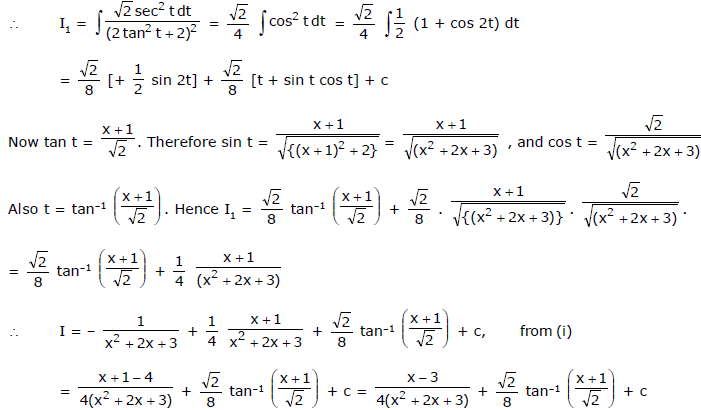Ex.51 If Im=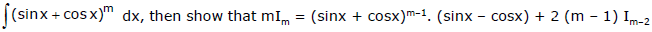Sol.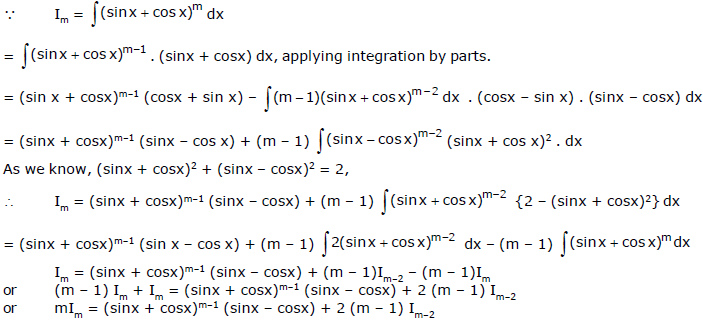Ex.52 If Im,n =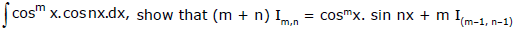Sol. We have, Im,n =cosm x.cosnx dx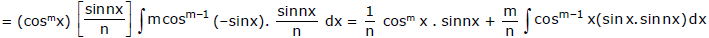As we have cos (n – 1) x = cos nx cos x + sin nx . sinx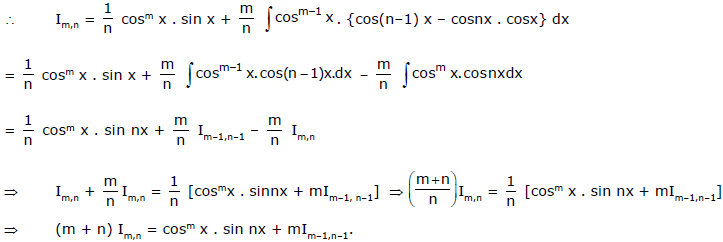Offer running on EduRev: Apply code STAYHOME200 to get INR 200 off on our premium plan EduRev Infinity!

,

,

,

,

,

,

,

,

,

,

,

,

,

,

,

,

,

,

,

,

,

,

,

,

,

,

,

;